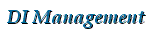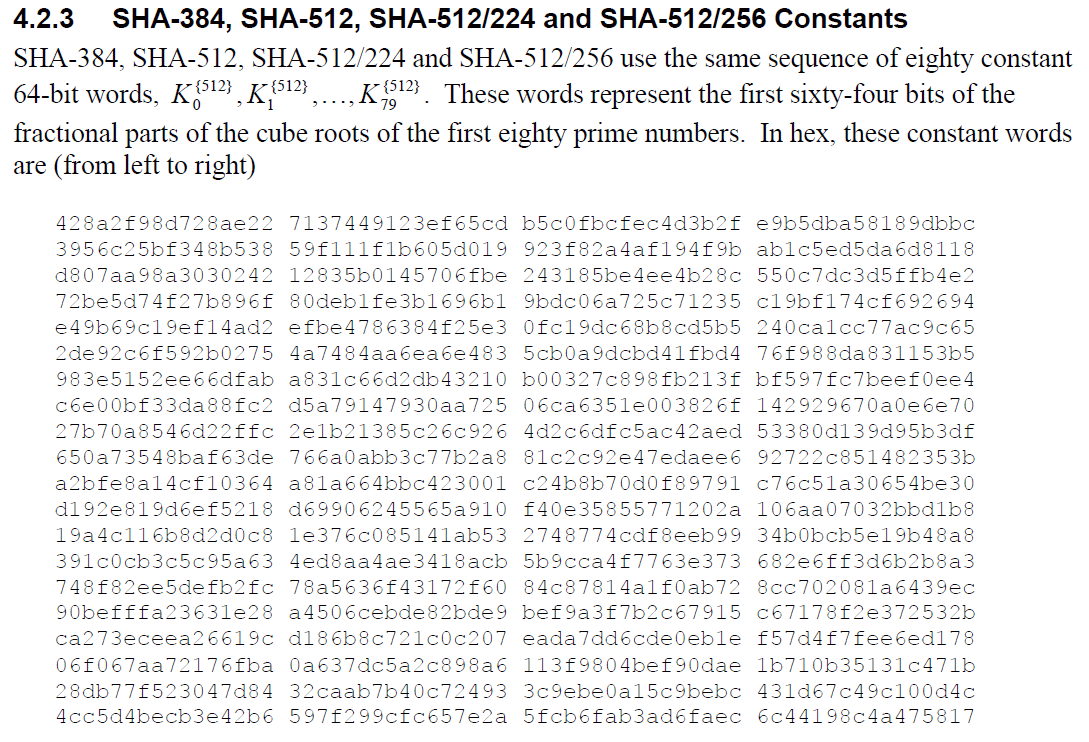DI Management Home > Mathematics > Computing a cube root in hexadecimal

#Computing a cube root in hexadecimal

This page shows how to compute the cube root of a number in hexadecimal format and describes the algorithm to find the exact digits. It includes source code in ANSI C that computes the first 64 bits of the fractional parts of the cube roots of the first eighty prime numbers. These are the constants used in FIPS PUB 180-4 for the SHA-384 and SHA-512 algorithms, and in a shorter 32-bit form, for the SHA-224 and SHA-256 algorithms.

## Finding the cube root

The algorithm to compute the cube root of a number works in a similar way to how you do long division by hand. We work through the input from left to right in groups of three digits at a time (we call this a triple). Each triple will give us one extra digit in our cube root.

In decimal (base 10), the largest triple we can have is $999$ and $\sqrt{999} \lt 10$, so the cube root of a triple is never bigger than one digit. This holds for any base (see Note 5 below).

We ignore decimal points in the calculation and just work with large integers. We can always put the decimal point back into the cube root afterwards. The input must be an exact multiple of three digits.

As a simple example, the cube root of 008 000 000 000 is 2000. We can shift the decimal points to get, for example, $\sqrt{8.000000000} = 2.000$ or $\sqrt{8000.000000} = 20.00$, provided we shift by three digits in the cube for every single digit in the root. The more trailing zeros we add to the input, the better precision we get in the root.

The cube root algorithm uses the identity $(a+b)^3 = a^3 + 3a^2b + 3ab^2 + b^3$. Roughly speaking, if $a$ was the best approximation we had to the cube root previously, and if $(a+b)$ is our next best estimate, then the "error" is given by $S = (a+b)^3 - a^3 = 3a^2b + 3ab^2 + b^3 = b(3a^2 + 3ab + b^2)$

## The algorithm

Algorithm: Compute the cube root of an integer in hexadecimal.
INPUT: An integer Z represented as a string str of 3k hexadecimal digits.
OUTPUT: The k-digit hexadecimal encoding of a, the greatest integer such that $a^3 \leq Z$.
1. Set $a \leftarrow 0$ and $R \leftarrow 0$
2. for each triple hhh of digits in str do
3.     Set $t \leftarrow$ decoded value of hhh
4.     Set $R \leftarrow R \times 16^3 + t$
5.     Find the greatest value of $d$ in the range $0\leq d \lt 16$ that satisfies the inequality $S \leq R$ where
$S = (3\times 16^2 \times a^2 + 3 \times 16 \times ad + d^2)d$
6.     Set $R \leftarrow R - S$ and $a \leftarrow 16a + d$
7. end for
8. return hex encoding of $a$.

To do the above algorithm in decimal, just replace all occurences of 16 with 10 and pass a string of decimal digits in blocks of three.

If at any stage we get $R=0$ at step (6) and all remaining input triples are zero, then we can stop and return the exact cube root. Otherwise we are dealing with an irrational number which goes on forever.

## The source code

The source code to do this is in hexcuberoots.c [zipped]. It uses our free BigDigits library to handle the large integers you need to get the required precision. Please feel free to use this code in your applications or research, just don't post it anywhere else or claim you wrote it.

Note that the 80th prime is 409 and $\sqrt{409} \lt 8$ so we can safely ignore the first digit of our calculation to obtain the fractional part of the cube root.

## The output

The output should look like this [full details]:

 1: frac_cbrt(2)=	428a2f98d728ae22
2: frac_cbrt(3)=	7137449123ef65cd
3: frac_cbrt(5)=	b5c0fbcfec4d3b2f
4: frac_cbrt(7)=	e9b5dba58189dbbc
...
80: frac_cbrt(409)=	6c44198c4a475817


You can compare the 64-bit fractional parts computed above with the values given in FIPS PUB 180-4:## Background notes and discussion

1. The use of the identity $(a+b)^3$ to compute an approximation to the cube root was first recorded by Fibonacci in the 13th Century. See the sample page on Finding Cube Roots from Fibonacci's De Practica Geometrie by Barnabas Hughes.
2. The Math Forum "Ask Dr. Math" gives some good examples of how to compute a cube root by hand at Cube Root by Hand and Cube Root Calculation, Explained.
3. Keith Vetter gives some sample TCL code to compute what he calls the "Long Square Root". Note that the equivalent identity for a square root is $(a+b)^2 = a^2 + 2ab + b^2$, and you can see that being used in the code.
4. Niel de Beaudrap (Neil?) gives a detailed explanation of how to find the cube root in binary in his answer on the Mathematics Stack Exchange.
5. Why is the integer cube root of a triple never more than one digit?

Suppose the base is $b$. The largest digit is $b-1$ (for example, for base $10$ the largest digit is $9$). The largest number $Z$ represented by the triple digits $ddd$ is given by $Z=(b-1)b^2+(b-1)b+(b-1) = b^3 -1 \lt b^3$ and it follows that $\sqrt{Z} \lt b$ and therefore the integer cube root can be represented by one digit. $\square$

## Acknowledgements

Thanks to Marc-Andre Deschenes for pointing out an error in the expression for $S$ in step 5 of the algorithm

## References

• [FIPS PUB 180-4] Secure Hash Standard (SHS), FIPS PUB 180-4, U.S. Department of Commerce/National Institute of Standards and Technology, March 2012, <pdf link>.